# The Meaning of PercentProblem: What fraction of each grid is shaded?

 Grid 1 Grid 2 Grid 3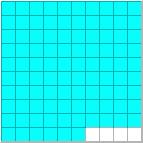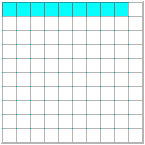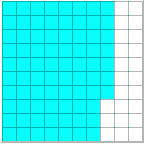Answer Answer Answer

Each grid above has 100 boxes. For each grid, the ratio of the number of shaded boxes to the total number of boxes can be represented as a fraction.

 Comparing Shaded Boxes to Total Boxes Grid Ratio Fraction 1 96 to 100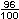2 9 to 1003 77 to 100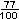We can represent these fractions as percents using the symbol %.= 96%= 9%= 77%Definition: A percent is a ratio whose second term is 100. Percent means parts per hundred. The word comes from the Latin phrase per centum, which means per hundred. In mathematics, we use the symbol % for percent.

Let's look at our comparison table again. This time the table includes percents.

 Comparing Shaded Boxes to Total Boxes Grid Ratio Fraction Percent 1 96 to 10096% 2 09 to 10009% 3 77 to 10077%

Let's look at some examples in which we are asked to convert between ratios, fractions, decimals and percents.

Example 1: Write each ratio as a fraction, a decimal, and a percent:   4 to 100,   63 to 100,   17 to 100

 Solution Ratio Fraction Decimal Percent 04 to 100.04 04% 63 to 100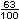.63 63% 17 to 100.17 17%

Example 2: Write each percent as a ratio, a fraction in lowest terms, and a decimal:   24%,   5%,   12.5%

 Solution Percent Ratio Fraction Decimal 24% 24 to 100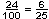.240 05% 05 to 100.050 12.5% 12.5 to 100.125

Example 3: Write each percent as a decimal:   91.2%,   4.9%,   86.75%

 Solution Percent Decimal 91.2% .9120 04.9% .0490 86.75% .8675

Summary: A percent is a ratio whose second term is 100. Percent means parts per hundred and we use the symbol % to represent it. In this lesson, we learned how to convert between ratios, fractions, decimals and percents.

### Exercises

Directions: Read each question below. Select your answer by clicking on its button. Feedback to your answer is provided in the RESULTS BOX. If you make a mistake, choose a different button.

 1.  Which of the following is equal to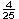? 1.6       8 to 100       16%       None of the above.       RESULTS BOX:
 2.  Which of the following is equal to 21.8%? 218 to 100       .218None of the above.       RESULTS BOX:
 3.  Which of the following is equal to 150% 15.0       1.50       .150       None of the above.       RESULTS BOX:
 4.  Which of the following is equal to .0179? 1.79%       17.9%       179%       None of the above.       RESULTS BOX:
 5.  Which of the following is equal to 56.28%? 56.28       5.628       .5628       None of the above.       RESULTS BOX: